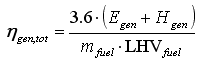# HOMER Pro 3.12

 Navigation: Glossary Generator Average Total EfficiencyType: Output Variable Units: % Symbol: ηgen,tot

The generator average total efficiency is the average of the generator's total efficiency over a year, defined as the electrical plus thermal energy out divided by fuel energy in. HOMER uses the following equation to calculate the average total efficiency:where: Egen = the generator's total annual electrical production [kWh/yr] Egen = the generator's total annual thermal production [kWh/yr] mfuel = the generator's total annual fuel consumption [kg/yr] LHVfuel = the lower heating value of the fuel [MJ/kg]

We use 3.6 in the equation above because 1 kWh = 3.6 MJ.

See also:

Generator Average Electrical Efficiency